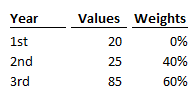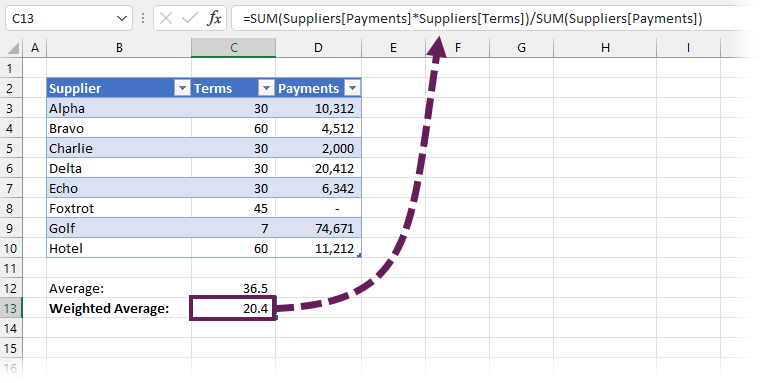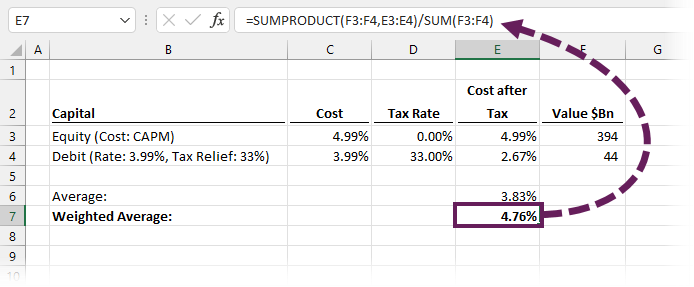Get our FREE VBA eBook of the 30 most useful Excel VBA macros.

Automate Excel so that you can save time and stop doing the jobs a trained monkey could do.

# Weighted Average in ExcelThe weighted average (also known as the weighted mean) is a calculation that provides an average where each value does not carry an equal impact on the final result. Using Excel’s AVERAGE function in these scenarios would give a misleading result. Thankfully, we can easily calculate the weighted average in Excel too.

Contents

## When to use a weighted average?

It’s not always easy to know when to calculate an average vs. a weighted average. Here are some examples to help you see where a weighted average is the correct calculation.

• In education, all assessments and exams do not have the same impact on the final grade. For example, at university, my final paper counted as 40% of my overall result, with other exams all having equal value. Therefore, we need to calculate the correct result by using a weighted average.
• Looking at average payment terms for suppliers can be a way to understand cash flow. For example, if a business has 10 suppliers with varying payment terms from 7 to 60 days, it may be tempting to use an average. However, if one supplier is 85% of total spend and only that supplier has 7-day terms, then it is necessary to use a weighted average. That one supplier will have a massive impact on the final result.
• A company may calculate its weighted average cost of capital, and use this as the discount rate to assess projects/investments. The idea is that if capital costs 4%, then we need projects to make a return larger than 4%. Otherwise, the cost of financing the project is more than the project returns, so it would be better not to invest at all.

The calculations above clearly demonstrate the importance of selecting the correct calculation. The average calculations would be mathematically accurate; however, they would be the wrong measure for decision-making.

## Basic calculation

Here is a basic scenario. For a qualification, 40% of Year 2 and 60% of Year 3 count towards the final grade. One student’s results are as follows, what is their final grade?To calculate a weighted average:

• Step 1: Each value is multiplied by the the corresponding weight
20 x 0% = 0
25 x 40% = 10
85 x 60% = 51
• Step 2: Calculate the sum of each item from Step 1
0 + 10 + 51 = 61
• Step 3: Divide the result from Step 2 by the SUM of total weights.
61 / (0% + 40% + 60%) = 61%

The final result is 61%## Weighted average in Excel

We can use simple functions to calculate the weighted average in Excel.

`=SUMPRODUCT(weights,values)/SUM(weights)`

Dynamic Array enabled Excel

`=SUM(weights*values)/SUM(weights)`

To use these formulas, we just need to insert the weights and values for our data. Let’s look at this with the 3 examples we introduced earlier.

### Weighted average to calculate exam results

In this scenario, 10 exams and one written paper contribute to the final result. The written paper is worth 40% of the final result; therefore, each exam is only worth 6% of the result.The formula in Cell C16 is:

`=SUMPRODUCT(D3:D13,C3:C13)/SUM(D3:D13)`

The SUMPRODUCT function achieves Steps 1 and 2 of the calculation; it multiplies each value with each corresponding weight and aggregates the total. Finally, step 3 calculates as the result of SUMPRODUCT divided by the SUM of the weights.

Exam results are a reasonably straightforward scenario to understand. The total weights add to 100%, so we can have a gut feeling about whether the total looks accurate.

### Weighted average to calculate average payment terms

In this scenario, we have one supplier on 7-day terms who is 85% of the cost, and then 7 other suppliers varying from 30 to 60 days.The values have been entered inside an Excel Table, and I am using a dynamic array enabled version of Excel. Therefore, I can use a calculation with only SUM functions.

The formula in Cell C13 is:

`=SUM(Suppliers[Payments]*Suppliers[Terms])/SUM(Suppliers[Payments])`

The first SUM function achieves Steps 1 and 2 (i.e., the multiplication and initial aggregation). Then in Step 3, the result is divided by the aggregated of the weights.

If we don’t have a dynamic array-enabled version of Excel, we can still use this method. Press Ctrl + Shift + Enter to confirm the formula. This will surround the formula in curly brackets, as shown below, and calculate the correct value in all versions of Excel.

`{=SUM(Suppliers[Payments]*Suppliers[Terms])/SUM(Suppliers[Payments])}`

### Weighted average cost of capital (WACC)

For this final example, we calculate a common business requirement: weighted average cost of capital in Excel.

Weighted average cost of capital (WACC) has the added complexity that the cost of debt is tax-deductible while return on equity is not. In practical terms, it means the published debt rate needs to be adjusted for tax before being included in the calculation.

The numbers below are based on an article from investopedia.com. The company’s equity of \$394B has a cost of 4.99%, while the debt of \$44B has a cost of 3.99%. The debt rate is adjusted for a 33% tax rate.The formula in Cell E7 is:

`=SUMPRODUCT(F3:F4,E3:E4)/SUM(F3:F4)`

This example demonstrates that in some scenarios, we may need to adjust values where there are factors affecting some numbers but not others. We want to calculate on a consistent basis.

## Common errors

Here are some common errors you might encounter when calculating weighted average in Excel:

• In the multiplication, the #VALUE! error will be returned if the ranges or arrays are of different sizes. This is true for the SUMPRODUCT or SUM approach.
• SUMPRODUCT and SUM both treat text as zeros, so if your ranges contain any text, it may not calculate the correct result

## Conclusion

There is no weighted average function in Excel, but with two simple functions, SUMPRODUCT and SUM, we can easily calculate it ourselves.Get our FREE VBA eBook of the 30 most useful Excel VBA macros.

Automate Excel so that you can save time and stop doing the jobs a trained monkey could do.

Don’t forget:

I’m guessing the examples in this post didn’t exactly meet your situation.  We all use Excel differently, so it’s impossible to write a post that will meet everybody’s needs.  By taking the time to understand the techniques and principles in this post (and elsewhere on this site) you should be able to adapt it to your needs.

But, if you’re still struggling you should:

1. Read other blogs, or watch YouTube videos on the same topic.  You will benefit much more by discovering your own solutions.
2. Ask the ‘Excel Ninja’ in your office.  It’s amazing what things other people know.
3. Ask a question in a forum like Mr Excel, or the Microsoft Answers Community.  Remember, the people on these forums are generally giving their time for free.  So take care to craft your question, make sure it’s clear and concise.  List all the things you’ve tried, and provide screenshots, code segments and example workbooks.
4. Use Excel Rescue, who are my consultancy partner.   They help by providing solutions to smaller Excel problems.

What next?
Don’t go yet, there is plenty more to learn on Excel Off The Grid.  Check out the latest posts:

## One thought on “Weighted Average in Excel”

1.Irfan A Khan says:

Thank you Mark.